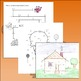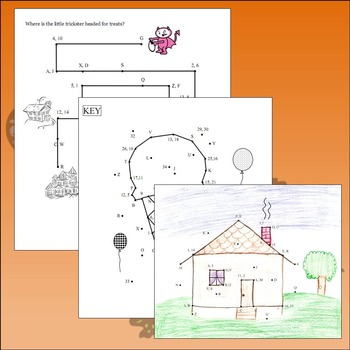# Solving Algebraic Equations BundleSubject
Resource Type
File Type
Zip (2 MB|20 pages)
Standards
\$15.00
Bundle
List Price:
\$22.75
You Save:
\$7.75
Products in this Bundle (7)

showing 1-5 of 7 products

• Bundle Description
• Standards

This bundle includes SEVEN Solving Algebraic Equations riddle/picture/maze worksheets, covering everything from simple one-step equations through multi-step inequalities.

Students will enjoy solving these worksheets as they enable them to complete riddles, pictures and a maze. The solution, in addition to being a fun alternative to a standard answer key, allows students to verify the accuracy of their calculations.

★★★  T  E  A  C  H  I  N  G    T  I  P  ★★★

Extra credit opportunities can be incorporated by allow students to strive for precision with the pictures and maze, as well as finishing the picture with ink, color, additional elements, etc. (see Student Sample in Preview)

★★★  Worksheets Included  ★★★

#110 - One-Step Equations Picture (Ice Cream Cone)

#112 - One-Step Equations (Mult/Div) Riddle

#114 - One-Step Equations (Fractions) Riddle

#120 - One and Two Step Equations Picture (House)

#122 - Two-Step Equations Riddle

#126 - Multi-Step Equation Maze

#132 - Multi-Step Inequalities Riddle

Solve linear equations and inequalities in one variable, including equations with coefficients represented by letters.
Solve linear equations in one variable.
Use variables to represent quantities in a real-world or mathematical problem, and construct simple equations and inequalities to solve problems by reasoning about the quantities.
Solve real-world and mathematical problems by writing and solving equations of the form 𝘹 + 𝘱 = 𝘲 and 𝘱𝘹 = 𝘲 for cases in which 𝘱, 𝘲 and 𝘹 are all nonnegative rational numbers.
Total Pages
20 pages